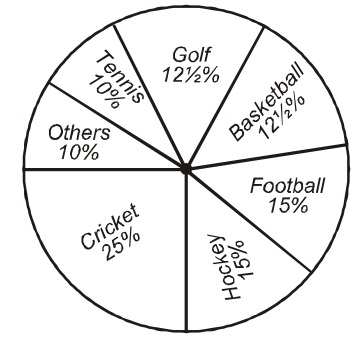## Pie chart

#### Data Interpretation

Direction: The pie chart, drawn here, shows the spending of a country on various sports during a particular year. Study the graph carefully and answer the questions that follow:1. The ratio of the total amount spent on football to that spent on hockey is:

1. Expenditure on the game of Football = 15% of total amount spent
Expenditure on the game of Hockey = 15% of total amount spent

##### Correct Option: B

Expenditure on the game of Football = 15% of total amount spent
Expenditure on the game of Hockey = 15% of total amount spent
Therefore, the ratio of the expenditure on the two game = 1 : 1

1. If the total amount spent on sports during the year was Rs. 30,00,000. The amount spent on cricket and hockey together was:

1. According to question the total amount spent = Rs. 30,00,000 = 100%
∴ Expenditure on the games of Cricket and Hockey = 25% + 15% = 40%

##### Correct Option: B

According to question the total amount spent = Rs. 30,00,000 = 100%
∴ Total Expenditure on the games of Cricket and Hockey = 25% + 15% = 40%
∴ The total amount spend on the games of Cricket and Hockey = 40% of 30,00,000 = 40 x 30,00,000/100 = 40 x 30,000 = Rs. 12,00,000

1. If the total amount spent on sports during the year was Rs. 1,20,00,000. how much was spent on basketball?

1. According to question, Total spent 100% = 1,20,00,000
According to graph,
∴ Expenditure on the game of Basketball = 121/2 %

##### Correct Option: D

According to question, Total spent 100% = 1,20,00,000
According to graph,
∴ Expenditure on the game of Basketball = 121/2 %
∴ The Amount spend on the game of Basketball = 121/2% of 1,20,00,000 = 25 x 1,20,00,000/100 = Rs. 15,00,000

1. Graph shows that the most popular game of the country is:

1. According to the graph, expenditure on cricket is the maximum.

##### Correct Option: C

According to the graph, expenditure on cricket is the maximum. Therefore cricket is the most popular game.

1. Out of the following the country spent the same amount on:

1. According to the graph, Hockey and Football are having the same percentage.
Expenditure on the game of Football = 15% of total amount spent
Expenditure on the game of Hockey = 15% of total amount spent

##### Correct Option: B

According to the graph, equal amount has been spent on Hockey and Football because the same percentage given in graph.
Expenditure on the game of Football = 15% of total amount spent
Expenditure on the game of Hockey = 15% of total amount spent